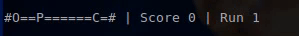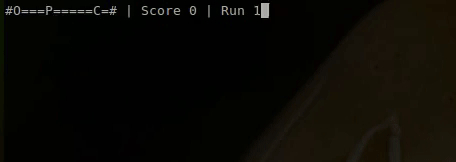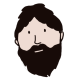## Teaching an AI to play a simple game using Q-learning

In this post I will walk you through how to teach a computer to master a simple video game using the q-learning reinforcement learning algorithm. We will implement the algorithm from scratch in Ruby without the use of external gems.

To enable us to illustrate the inner workings of the algorithm we will be teaching it to play a very simple 1 dimensional game. This algorithm can however can easily be applied to much more complex games.

## The game

Our game is a “catch the cheese” console game where the player P must move to catch the cheese C without falling into the pit O. The player gets one point of each cheese he finds and minus one point for every time he falls into the pit. The game ends if the user gets either 5 points or -5 points. This gif shows a human player playing the game.Note to simplify the game the position of the pit O and cheese C are always the same. You can find the Ruby source code of the game and the implementation of the learning algorithm in this repository.

## Q-learning algorithm

The Q-learning algorithm is a reinforcement learning algorithm. Reinforcement learning algorithms are a set of machine learning algorithms inspired by behavioral psychology. The basic premise is that you teach the algorithm to take certain actions based on prior experience by rewarding or punishing actions. Similar to teaching a dog to sit by giving it treats for good behavior.

The q-learning algorithm works by keeping a table of all possible game states and all possible player actions for these states. For each pair of game state S and player action the table contains a numerical value Q representing the possible reward for taking the action A at the state S. This means that to optimize our total reward our AI can, for a specific state, simply pick the action that has the largest potential reward from the table.

Consider our simple game from above. The game has a total of 12 possible states based on the position of the player (remember the position of the pit and cheese are static) and for each state the player can take two actions either go left or go right.

This gives us a Q table like this – note this is just an example of how a learned Q table could look for our game, the values in our actual Q table may differ:

States Action: Left Action: Right
0 0.2 0.6
1 -0.6 0.6
2  -0.4 0.5
3  -0.2 0.3
4  -0.1 0.3
5  0 0.2
6  0 0.5
7  0.1 0.6
8  0.3  0.8
9  0.2  1
10  1  0.5
11  0.6  0.2

As you can see the Q table indicates that when close to the pit taking the left action may have a negative reward, while going right, closer to the cheese, may  have a positive reward,

The Q table when initialized is initialized to random values, this is done since the AI yet does not know anything about the game. To learn how to play your game we must devise an algorithm for updating the Q table based on experience.

We do this as follows:

Step 1: Initialize Q table with random values
Step 2: While playing the game execute the following loop
Step 2.a: Generate random number between 0 and 1 – if number is larger than the threshold e select random action, otherwise select action with the highest possible reward based on state and Q-table
Step 2.b: Take action from step 2.a
Step 2.c: Observe reward r after taking action
Step 2.d: Update Q table based on the reward r using the formula

$\displaystyle Q(s_{t},a_{t})\leftarrow \underbrace {Q(s_{t},a_{t})} _{\rm {old~value}}+\underbrace {\alpha } _{\rm {learning~rate}}\cdot \left(\overbrace {\underbrace {r_{t}} _{\rm {reward}}+\underbrace {\gamma } _{\rm {discount~factor}}\cdot \underbrace {\max _{a}Q(s_{t+1},a)} _{\rm {estimate~of~optimal~future~value}}} ^{\rm {learned~value}}-\underbrace {Q(s_{t},a_{t})} _{\rm {old~value}}\right)$

As you can see the update to the Q table will use the newly learned reward information for the current state and action, as well as information about future actions without having to traverse all possible future actions, but by simply using the Q table. This makes Q learning a rather fast learning algorithm, but also means that it does have some random behavior in the beginning stages so don’t expect your AI to be perfect until after a couple of playthroughs.

For more details on Q-learning go here.

### Implementing a Q-Learning AI player class

Our game normally takes an instance of the human player class as the player object. The implementation of the human player class is shown below. The player class implements two functions, an constructor and a get_input function.

The get_input function is called once on every iteration of the game loop, and returns the player direction based on keyboard input as follows.

require 'io/console'
class Player
attr_accessor :x
def initialize
@x = 0
end

def get_input
input = STDIN.getch
if input == 'a'
return :left
elsif input == 'd'
return :right
elsif input == 'q'
exit
end
return :nothing
end
end


To build a Q-learning AI player class we will implement a new player class that holds the q-table and implements the get_input function based on the algorithm outlined above.

We define our new class and its constructor as follows below. Note we have a new attribute game defined for this player. This is needed since the q-learning algorithm needs to reference the game score in order to update the Q table. Additionally in our constructor we define attributes for each of the learning parameters outlined in the algorithm above and initialize our random generator.

class QLearningPlayer
attr_accessor :x, :game

def initialize
@x = 0
@actions = [:left, :right]
@first_run = true

@learning_rate = 0.2
@discount = 0.9
@epsilon = 0.9

@r = Random.new
end

Next we define a function to initialize our Q table with random values. The number of states is the map_size of the game, each state will simply be the position of the player.

def initialize_q_table
# Initialize q_table states by actions
@q_table = Array.new(@game.map_size){ Array.new(@actions.length) }

# Initialize to random values
@game.map_size.times do |s|
@actions.length.times do |a|
@q_table[s][a] = @r.rand
end
end
end

Finally we implement the get_input function as follows. First we pause for 0.05 seconds to enable us to follow along with the graphics of the game for the AI player.

Next we we check if this is the first run, if so we initialize the Q table (Step 1)

If this is not the first run we evaluate what happened in the game since the last time we were asked for input to determine our reward r for the Q learning algorithm. If the score of the game increased we set our reward to 1 and if it decreased we set our reward to -1, if the score didn’t change our reward is 0 (Step 2.c).

Then we set our outcome state to the current state of the game (in our case the position of the player) and update the Q table following the equation (Step 2.d):

$\displaystyle Q(s_{t},a_{t})\leftarrow \underbrace {Q(s_{t},a_{t})} _{\rm {old~value}}+\underbrace {\alpha } _{\rm {learning~rate}}\cdot \left(\overbrace {\underbrace {r_{t}} _{\rm {reward}}+\underbrace {\gamma } _{\rm {discount~factor}}\cdot \underbrace {\max _{a}Q(s_{t+1},a)} _{\rm {estimate~of~optimal~future~value}}} ^{\rm {learned~value}}-\underbrace {Q(s_{t},a_{t})} _{\rm {old~value}}\right)$

def get_input
# Pause to make sure humans can follow along
sleep 0.05

if @first_run
# If this is first run initialize the Q-table
initialize_q_table
@first_run = false
else
# If this is not the first run
# Evaluate what happened on last action and update Q table
# Calculate reward
r = 0 # default is 0
if @old_score < @game.score
r = 1 # reward is 1 if our score increased
elsif @old_score > @game.score
r = -1 # reward is -1 if our score decreased
end

# Our new state is equal to the player position
@outcome_state = @x
@q_table[@old_state][@action_taken_index] = @q_table[@old_state][@action_taken_index] + @learning_rate * (r + @discount * @q_table[@outcome_state].max - @q_table[@old_state][@action_taken_index])
end


After updating the Q table based on our last step, we now capture the old score and old state to use on our next run.

We then choose a new action either randomly or based on the Q table depending on epsilon e (Step 2.a) and return that action (Step 2.b).

  # Capture current state and score
@old_score = @game.score
@old_state = @x

# Chose action based on Q value estimates for state
if @r.rand > @epsilon
# Select random action
@action_taken_index = @r.rand(@actions.length).round
else
# Select based on Q table
s = @x
@action_taken_index = @q_table[s].each_with_index.max
end

# Take action
return @actions[@action_taken_index]
end


And that completes our implementation of the Q-learning algorithm. You can see the full source code for the algorithm and the game here.

## Running the algorithm

With our Q-learning player created we spin up the game and let our new player play 10 runs of the games.As you can see on the first run the player is trying all kinds of different things and moves back and forward without aim. This is due to the random initialization of the Q-table. However once the player has gotten a few points and fell into a few pits, it quickly learns to avoid the pits and go straight for the cheese.

On run 7 and 8 the AI player comes very close to the perfect solution, which is going straight for the cheese in 35 moves. However on run 9 and 10 it takes 39 moves to win the game. This is due to the epsilon factor ethat sometimes causes the algorithm to make a random move rather than the optimal move. This randomness is required to ensure the algorithm can properly explore the entire game and won’t get stuck at local optimums.

## Next steps …

This post showed  how to apply q-learning to teach an AI to play a simple game. As you can imagine as the complexity of the games increase the size of the Q table explodes. One way of avoiding this is to replace the Q table by a neural network.

This approach was explored in the paper Playing Atari with Deep Reinforcement Learning by researchers at DeepMind Technologies. In this paper they successfully trained Q-learning with a neural network Q table to play Space Invaders, Pong, Q*bert and other Atari 2600 games.

In our next reinforcement learning post we will expand our game to make it 2D and we will try to train a new AI player using Q-learning and a neural network to master the game. Stay tuned and sign-up for the newsletter to make sure you won’t miss it.

•Bobby Jack says:

The perfect solution is actually 35 moves

•Soren D says:

You’re absolutely right! Post have been updated to reflect this comment.
Thanks for commenting 🙂

•NicWagenaar says:

Came upon this post through the ruby weekly newsletter, then started reading the rest of the blog. Really good stuff! Thanks for taking a very practical approach to AI and ML, has really helped me get started in those areas. Looking forward to part two of this post!

•Soren D says:

Thanks! It’s great to know people are finding this useful. I’ll have part two up within a week or so.